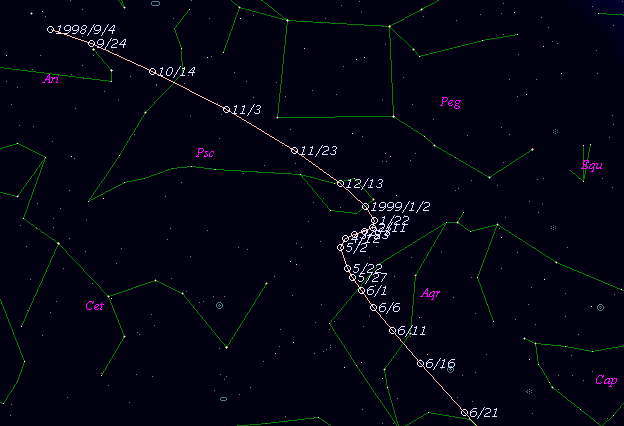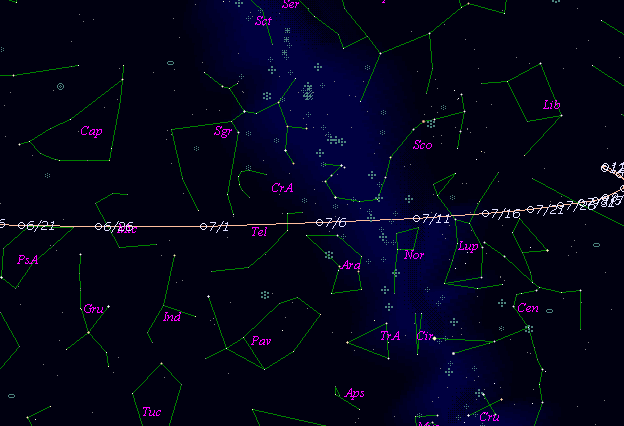# \$B%j%K%"WB@1(B

C/1998 T1 ( LINEAR )###\$B%W%m%U%#!<%k(B

 \$BH/8+F|(B 1998\$BG/(B10\$B7n(B2\$BF|(B \$BH/8+8wEY(B 17.6\$BEy(B \$BH/8+ Lincoln Laboratory Near-Earth Asteroid Research project

###\$B###\$B50F;MWAG(B

```   The following improved orbital elements by Kenji Muraoka, are
from 321 observations 1998 Oct. 2 to 1999 Dec. 27, perturbations
by 9 Planets, Moon and 5 minor planets were taken into account.
The mean residual is +/- 0.75 arc seconds.

Epoch  =  1999 July  1.0  TT       JDT = 2451360.5
T  =  1999 June 25.25150       +/- 0.00006 (m.e.) TT
Peri. =  226.35289                +/- 0.00013
Node  =  153.35713                +/- 0.00014   (2000.0)
Incl. =  170.15984                +/- 0.00002
q  =    1.4677240              +/- 0.0000004 AU
e  =    0.9991009              +/- 0.0000021
1/a  =   +0.0006126              +/- 0.0000014 1/AU
orig. 1/a  =   +0.000558
fut.  1/a  =   +0.001457
```

###\$B@1?^(B###\$B8wEYJQ2=(B

```        m1 = 8.4 + 5 log\$B&\$(B + 10.0 log r
```##### \$B50F;MWAG\$OB<2,7r<#;a\$N7W;;\$K\$h\$k\$b\$N\$G\$9!#(B \$B@1?^\$O(B StellaNavigator Ver.2.0 for Windows (\$B%"%9%H%m%"!<%D(B \$BJTCx(B / \$B%"%9%-!<=PHG6I4)(B) \$B\$G:n@.\$7\$?\$b\$N\$G\$9!#(B \$B8wEY%0%i%U\$O(BComet for Windows\$B\$G:n@.\$7\$?\$b\$N\$G\$9!#(B